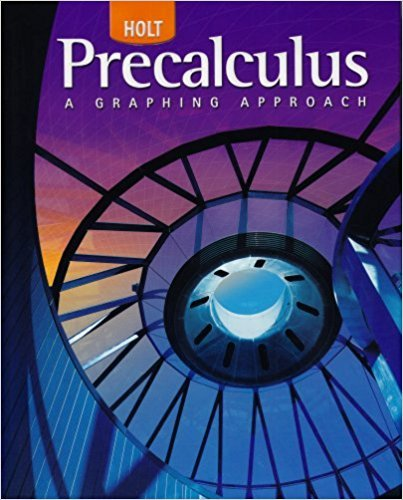×
×

# Solutions for Chapter 11.4: Analytic Geometry## Full solutions for Precalculus | 1st Edition

ISBN: 9780030416477Solutions for Chapter 11.4: Analytic Geometry

Solutions for Chapter 11.4
4 5 0 250 Reviews
13
0
##### ISBN: 9780030416477

This expansive textbook survival guide covers the following chapters and their solutions. Chapter 11.4: Analytic Geometry includes 27 full step-by-step solutions. This textbook survival guide was created for the textbook: Precalculus, edition: 1. Since 27 problems in chapter 11.4: Analytic Geometry have been answered, more than 26196 students have viewed full step-by-step solutions from this chapter. Precalculus was written by and is associated to the ISBN: 9780030416477.

Key Calculus Terms and definitions covered in this textbook
• Boxplot (or box-and-whisker plot)

A graph that displays a five-number summary

• Elements of a matrix

See Matrix element.

• Equally likely outcomes

Outcomes of an experiment that have the same probability of occurring.

• Factor

In algebra, a quantity being multiplied in a product. In statistics, a potential explanatory variable under study in an experiment, .

• Frequency (in statistics)

The number of individuals or observations with a certain characteristic.

• Grapher or graphing utility

Graphing calculator or a computer with graphing software.

• Independent variable

Variable representing the domain value of a function (usually x).

• Jump discontinuity at x a

limx:a - ƒ1x2 and limx:a + ƒ1x2 exist but are not equal

See Polynomial function in x.

• n factorial

For any positive integer n, n factorial is n! = n.(n - 1) . (n - 2) .... .3.2.1; zero factorial is 0! = 1

• Plane in Cartesian space

The graph of Ax + By + Cz + D = 0, where A, B, and C are not all zero.

• Real zeros

Zeros of a function that are real numbers.

• Reduced row echelon form

A matrix in row echelon form with every column that has a leading 1 having 0’s in all other positions.

• Remainder polynomial

See Division algorithm for polynomials.

• Resolving a vector

Finding the horizontal and vertical components of a vector.

• Sample space

Set of all possible outcomes of an experiment.

• Sequence

See Finite sequence, Infinite sequence.

• Variable

A letter that represents an unspecified number.

• Vertical line

x = a.

• x-axis

Usually the horizontal coordinate line in a Cartesian coordinate system with positive direction to the right,.

×